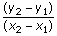## Definition Of Slope

Slope is the measure of steepness of a line.

Slope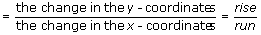.

Slope of a curve: The slope of a curve is the slope of a line tangent to a particular point on the graph of the curve.
Slope-intercept form: An equation of the form y = mx + b, where m is the slope and b is the y-intercept.

### Example of Slope

Slope of the line shown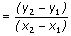.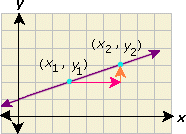### Solved Example on Slope

#### Ques: ABCD is a rectangle. The vertices of A are (- 7, - 5). Find the slope of the diagonal AC if the coordinate axes are the lines of symmetry for the rectangle shown.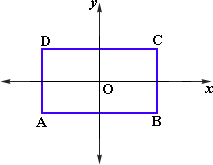##### Choices:

A. 5/7
B. 5/6
C. 7/5
D. 7/8
Step 3: Slope of AC is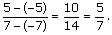[Slope of line passing through (x1, y1) and (x2, y2) is .]